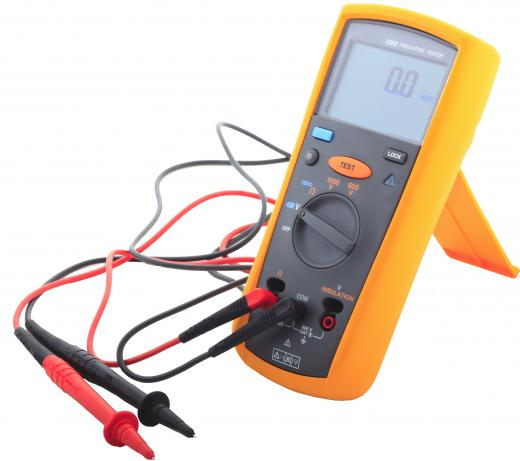Technology
Fact Checked

# What Is Involved in Multimeter Calibration?

Geisha A. Legazpi
Geisha A. Legazpi

Multimeter calibration involves making measurements and adjustments on different measurement modes of a multimeter or multitester. The most common modes of measurements are alternating current/direct current (AC/DC) voltage, AC/DC current, and DC resistance measurements. There are different multimeter calibration equipment and setups that serve as the calibration reference, depending on the accuracy required.

Digital multimeters usually have built-in processors that handle measurements using stable voltage references. Unlike digital multimeters, analog multimeters need to be calibrated to maintain accuracy. Analog multimeters usually have adjustable resistors called trimmer resistors that may be used to compensate for different conditions.A digital multimeter is a tool that can measure amps, volts, and ohms.

Electrical and electronic properties may change over repeated use of the multimeter. Different factors, such as resistors, meter movement sensitivity, and battery voltage, may affect the accuracy of multimeter readings. Some analog multimeters also use an internal battery for voltage and current measurements.

Different measurement ranges are checked in multimeter calibration. In a multimeter that uses a meter movement with 50 microamperes full scale, the manufacturer labels the DC full scale with 10, 50, 250, and 500 volts direct current (VDC). In this case, there are four range selector positions that measure DC voltage.

The needed total series resistance with a meter movement of 1 kilo-ohm (k-ohm) can be computed. At 10 VDC full scale, the current into the voltmeter needs to be 50 microamperes full scale and the total resistance involved is 10 volts (V)/50 microamperes, or 200 k-ohm. The total resistance is 200 k-ohm, thus the needed series resistance is 200 k-ohm less 1 k-ohm, or 199 k-ohm. Calibration is a matter of using a fixed resistance and an adjustable resistance to approximate 199 k-ohm. A typical example could be 180 k-ohm fixed resistance in series with a 20 k-ohm trimmer resistor for the 10 VDC range.

For AC multimeter calibration, the circuit is the same as for the DC multimeter circuit with added rectifier circuit. The diodes in the rectifier circuit convert the AC into proportional DC half- or full-wave pulses that are filtered and limited to drive the meter movement. With the right selection of series resistance, the readings are similar to that of a true root mean square (RMS) multimeter.

In DC ammeters, which measure DC currents, the meter movements are parallel with very low resistances. Calibration is usually done by adjusting a resistance in series with the meter movement. When a 50-microampere meter movement is parallel with a 0.1-ohm resistor, a full scale of 50 microampere corresponds to a small voltage of 0.05 VDC across the ammeter. This corresponds to a current of 0.5 ampere (A), and the full-scale label is then 500.05 milliamperes (mA).

## You might also Like